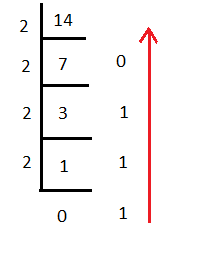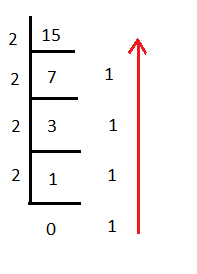### Decimal to binary conversion program in java

In this core java programming tutorial we will write a program to perform Decimal to binary conversion in java.

Decimal to binary conversion program in java.

>decimal 14 = binary 1110
Let’s understand by diagram how we calculate binary equivalent of decimal number.
divide 14 by 2, remainder is 0, result is 7.
divide 7 by 2, remainder is 1, result is 3.
divide 3 by 2, remainder is 1, result is 1.
divide 1 by 2, remainder is 1, result is 0.
Read remainders from bottom to up, that’s binary number for us.>decimal 15 = binary 1111
Let’s understand by diagram how we calculate binary equivalent of decimal number.
divide 15 by 2, remainder is 1, result is 7.
divide 7 by 2, remainder is 1, result is 3.
divide 3 by 2, remainder is 1, result is 1.
divide 1 by 2, remainder is 1, result is 0.
Read remainders from bottom to up, that’s binary number for us.Full Program/SourceCode/ example to perform Decimal to binary conversion in java>
 /** Copyright (c), AnkitMittal www.JavaMadeSoEasy.com */ public class DecimalToBinaryConversionExample {           public static void main(String...args) {                          int decimal=15;              System.out.println("decimal "+decimal+" = binary " + decimalToBinaryConverter(decimal));                    decimal=14;              System.out.println("decimal "+decimal+" = binary " + decimalToBinaryConverter(decimal));      }              /*           * method converts decimal number to binary.           */      public static String decimalToBinaryConverter(int decimal) {       if (decimal == 0) {           return "0"; //binary of decimal(0) is 0 only.       }       String binary = "";       while (decimal > 0) {         binary = (decimal % 2) + binary;           decimal = decimal / 2;       }       return binary;      }      } /*OUTPUT decimal 15 = binary 1111 decimal 14 = binary 1110 */

So in this core java programming tutorial we wrote a program on how to perform Decimal to binary conversion in java.

Having any doubt? or you you liked the tutorial! Please comment in below section.